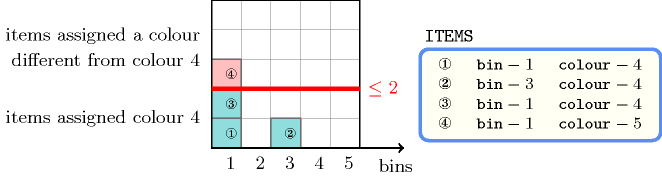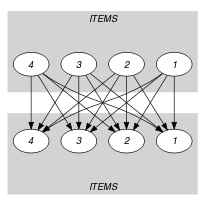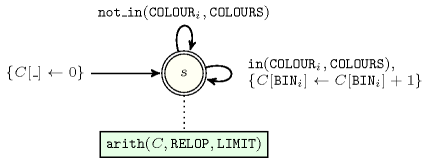## 5.34. assign_and_counts

Origin

N. Beldiceanu

Constraint

$\mathrm{𝚊𝚜𝚜𝚒𝚐𝚗}_\mathrm{𝚊𝚗𝚍}_\mathrm{𝚌𝚘𝚞𝚗𝚝𝚜}\left(\mathrm{𝙲𝙾𝙻𝙾𝚄𝚁𝚂},\mathrm{𝙸𝚃𝙴𝙼𝚂},\mathrm{𝚁𝙴𝙻𝙾𝙿},\mathrm{𝙻𝙸𝙼𝙸𝚃}\right)$

Arguments
 $\mathrm{𝙲𝙾𝙻𝙾𝚄𝚁𝚂}$ $\mathrm{𝚌𝚘𝚕𝚕𝚎𝚌𝚝𝚒𝚘𝚗}\left(\mathrm{𝚟𝚊𝚕}-\mathrm{𝚒𝚗𝚝}\right)$ $\mathrm{𝙸𝚃𝙴𝙼𝚂}$ $\mathrm{𝚌𝚘𝚕𝚕𝚎𝚌𝚝𝚒𝚘𝚗}\left(\mathrm{𝚋𝚒𝚗}-\mathrm{𝚍𝚟𝚊𝚛},\mathrm{𝚌𝚘𝚕𝚘𝚞𝚛}-\mathrm{𝚍𝚟𝚊𝚛}\right)$ $\mathrm{𝚁𝙴𝙻𝙾𝙿}$ $\mathrm{𝚊𝚝𝚘𝚖}$ $\mathrm{𝙻𝙸𝙼𝙸𝚃}$ $\mathrm{𝚍𝚟𝚊𝚛}$
Restrictions
 $\mathrm{𝚛𝚎𝚚𝚞𝚒𝚛𝚎𝚍}$$\left(\mathrm{𝙲𝙾𝙻𝙾𝚄𝚁𝚂},\mathrm{𝚟𝚊𝚕}\right)$ $\mathrm{𝚍𝚒𝚜𝚝𝚒𝚗𝚌𝚝}$$\left(\mathrm{𝙲𝙾𝙻𝙾𝚄𝚁𝚂},\mathrm{𝚟𝚊𝚕}\right)$ $\mathrm{𝚛𝚎𝚚𝚞𝚒𝚛𝚎𝚍}$$\left(\mathrm{𝙸𝚃𝙴𝙼𝚂},\left[\mathrm{𝚋𝚒𝚗},\mathrm{𝚌𝚘𝚕𝚘𝚞𝚛}\right]\right)$ $\mathrm{𝚁𝙴𝙻𝙾𝙿}\in \left[=,\ne ,<,\ge ,>,\le \right]$
Purpose

Given several items (each of them having a specific colour that may not be initially fixed), and different bins, assign each item to a bin, so that the total number $n$ of items of colour $\mathrm{𝙲𝙾𝙻𝙾𝚄𝚁𝚂}$ in each bin satisfies the condition $n\mathrm{𝚁𝙴𝙻𝙾𝙿}\mathrm{𝙻𝙸𝙼𝙸𝚃}$.

Example
$\left(\begin{array}{c}〈4〉,\hfill \\ 〈\begin{array}{cc}\mathrm{𝚋𝚒𝚗}-1\hfill & \mathrm{𝚌𝚘𝚕𝚘𝚞𝚛}-4,\hfill \\ \mathrm{𝚋𝚒𝚗}-3\hfill & \mathrm{𝚌𝚘𝚕𝚘𝚞𝚛}-4,\hfill \\ \mathrm{𝚋𝚒𝚗}-1\hfill & \mathrm{𝚌𝚘𝚕𝚘𝚞𝚛}-4,\hfill \\ \mathrm{𝚋𝚒𝚗}-1\hfill & \mathrm{𝚌𝚘𝚕𝚘𝚞𝚛}-5\hfill \end{array}〉,\le ,2\hfill \end{array}\right)$

Figure 5.34.1 shows the solution associated with the example. The items and the bins are respectively represented by little squares and by the different columns. Each little square contains the value of the $\mathrm{𝚔𝚎𝚢}$ attribute of the item to which it corresponds. The items for which the colour attribute is equal to 4 are located under the thick line.

##### Figure 5.34.1. Assignment of the items to the binsThe $\mathrm{𝚊𝚜𝚜𝚒𝚐𝚗}_\mathrm{𝚊𝚗𝚍}_\mathrm{𝚌𝚘𝚞𝚗𝚝𝚜}$ constraint holds since for each used bin (i.e., namely bins 1 and 3) the number of assigned items for which the colour attribute is equal to 4 is less than or equal to the limit 2.

Typical
 $|\mathrm{𝙲𝙾𝙻𝙾𝚄𝚁𝚂}|>0$ $|\mathrm{𝙸𝚃𝙴𝙼𝚂}|>1$ $\mathrm{𝚛𝚊𝚗𝚐𝚎}$$\left(\mathrm{𝙸𝚃𝙴𝙼𝚂}.\mathrm{𝚋𝚒𝚗}\right)>1$ $\mathrm{𝚁𝙴𝙻𝙾𝙿}\in \left[<,\le \right]$ $\mathrm{𝙻𝙸𝙼𝙸𝚃}>0$ $\mathrm{𝙻𝙸𝙼𝙸𝚃}<|\mathrm{𝙸𝚃𝙴𝙼𝚂}|$
Symmetries
• Items of $\mathrm{𝙲𝙾𝙻𝙾𝚄𝚁𝚂}$ are permutable.

• Items of $\mathrm{𝙸𝚃𝙴𝙼𝚂}$ are permutable.

• All occurrences of two distinct values of $\mathrm{𝙸𝚃𝙴𝙼𝚂}.\mathrm{𝚋𝚒𝚗}$ can be swapped; all occurrences of a value of $\mathrm{𝙸𝚃𝙴𝙼𝚂}.\mathrm{𝚋𝚒𝚗}$ can be renamed to any unused value.

Arg. properties
• Contractible wrt. $\mathrm{𝙸𝚃𝙴𝙼𝚂}$ when $\mathrm{𝚁𝙴𝙻𝙾𝙿}\in \left[<,\le \right]$.

• Extensible wrt. $\mathrm{𝙸𝚃𝙴𝙼𝚂}$ when $\mathrm{𝚁𝙴𝙻𝙾𝙿}\in \left[\ge ,>\right]$.

Usage

Some persons have pointed out that it is impossible to use constraints such as $\mathrm{𝚊𝚖𝚘𝚗𝚐}$, $\mathrm{𝚊𝚝𝚕𝚎𝚊𝚜𝚝}$, $\mathrm{𝚊𝚝𝚖𝚘𝚜𝚝}$, $\mathrm{𝚌𝚘𝚞𝚗𝚝}$, or $\mathrm{𝚐𝚕𝚘𝚋𝚊𝚕}_\mathrm{𝚌𝚊𝚛𝚍𝚒𝚗𝚊𝚕𝚒𝚝𝚢}$ if the set of variables is not initially known. However, this is for instance required in practice for some timetabling problems.

Keywords
Derived Collection
$\mathrm{𝚌𝚘𝚕}\left(\mathrm{𝚅𝙰𝙻𝚄𝙴𝚂}-\mathrm{𝚌𝚘𝚕𝚕𝚎𝚌𝚝𝚒𝚘𝚗}\left(\mathrm{𝚟𝚊𝚕}-\mathrm{𝚒𝚗𝚝}\right),\left[\mathrm{𝚒𝚝𝚎𝚖}\left(\mathrm{𝚟𝚊𝚕}-\mathrm{𝙲𝙾𝙻𝙾𝚄𝚁𝚂}.\mathrm{𝚟𝚊𝚕}\right)\right]\right)$
Arc input(s)

$\mathrm{𝙸𝚃𝙴𝙼𝚂}$ $\mathrm{𝙸𝚃𝙴𝙼𝚂}$

Arc generator
$\mathrm{𝑃𝑅𝑂𝐷𝑈𝐶𝑇}$$↦\mathrm{𝚌𝚘𝚕𝚕𝚎𝚌𝚝𝚒𝚘𝚗}\left(\mathrm{𝚒𝚝𝚎𝚖𝚜}\mathtt{1},\mathrm{𝚒𝚝𝚎𝚖𝚜}\mathtt{2}\right)$

Arc arity
Arc constraint(s)
$\mathrm{𝚒𝚝𝚎𝚖𝚜}\mathtt{1}.\mathrm{𝚋𝚒𝚗}=\mathrm{𝚒𝚝𝚎𝚖𝚜}\mathtt{2}.\mathrm{𝚋𝚒𝚗}$
Graph class
 $•$$\mathrm{𝙰𝙲𝚈𝙲𝙻𝙸𝙲}$ $•$$\mathrm{𝙱𝙸𝙿𝙰𝚁𝚃𝙸𝚃𝙴}$ $•$$\mathrm{𝙽𝙾}_\mathrm{𝙻𝙾𝙾𝙿}$

Sets
$\begin{array}{c}\mathrm{𝖲𝖴𝖢𝖢}↦\hfill \\ \left[\begin{array}{c}\mathrm{𝚜𝚘𝚞𝚛𝚌𝚎},\hfill \\ \mathrm{𝚟𝚊𝚛𝚒𝚊𝚋𝚕𝚎𝚜}-\mathrm{𝚌𝚘𝚕}\left(\begin{array}{c}\mathrm{𝚅𝙰𝚁𝙸𝙰𝙱𝙻𝙴𝚂}-\mathrm{𝚌𝚘𝚕𝚕𝚎𝚌𝚝𝚒𝚘𝚗}\left(\mathrm{𝚟𝚊𝚛}-\mathrm{𝚍𝚟𝚊𝚛}\right),\hfill \\ \mathrm{𝚒𝚝𝚎𝚖}\left(\mathrm{𝚟𝚊𝚛}-\mathrm{𝙸𝚃𝙴𝙼𝚂}.\mathrm{𝚌𝚘𝚕𝚘𝚞𝚛}\right)\right]\hfill \end{array}\right)\hfill \end{array}\right]\hfill \end{array}$

Constraint(s) on sets
$\mathrm{𝚌𝚘𝚞𝚗𝚝𝚜}$$\left(\mathrm{𝚅𝙰𝙻𝚄𝙴𝚂},\mathrm{𝚟𝚊𝚛𝚒𝚊𝚋𝚕𝚎𝚜},\mathrm{𝚁𝙴𝙻𝙾𝙿},\mathrm{𝙻𝙸𝙼𝙸𝚃}\right)$
Graph model

We enforce the $\mathrm{𝚌𝚘𝚞𝚗𝚝𝚜}$ constraint on the colour of the items that are assigned to the same bin.

Parts (A) and (B) of Figure 5.34.2 respectively show the initial and final graph associated with the Example slot. The final graph consists of the following two connected components:

• The connected component containing six vertices corresponds to the items that are assigned to bin 1.

• The connected component containing two vertices corresponds to the items that are assigned to bin 3.

##### Figure 5.34.2. Initial and final graph of the $\mathrm{𝚊𝚜𝚜𝚒𝚐𝚗}_\mathrm{𝚊𝚗𝚍}_\mathrm{𝚌𝚘𝚞𝚗𝚝𝚜}$ constraint(a) (b)

The $\mathrm{𝚊𝚜𝚜𝚒𝚐𝚗}_\mathrm{𝚊𝚗𝚍}_\mathrm{𝚌𝚘𝚞𝚗𝚝𝚜}$ constraint holds since for each set of successors of the vertices of the final graph no more than two items take colour 4.

Automaton

Figure 5.34.3 depicts the automaton associated with the $\mathrm{𝚊𝚜𝚜𝚒𝚐𝚗}_\mathrm{𝚊𝚗𝚍}_\mathrm{𝚌𝚘𝚞𝚗𝚝𝚜}$ constraint. To each $\mathrm{𝚌𝚘𝚕𝚘𝚞𝚛}$ attribute ${\mathrm{𝙲𝙾𝙻𝙾𝚄𝚁}}_{i}$ of the collection $\mathrm{𝙸𝚃𝙴𝙼𝚂}$ corresponds a 0-1 signature variable ${S}_{i}$. The following signature constraint links ${\mathrm{𝙲𝙾𝙻𝙾𝚄𝚁}}_{i}$ and ${S}_{i}$: ${\mathrm{𝙲𝙾𝙻𝙾𝚄𝚁}}_{i}\in \mathrm{𝙲𝙾𝙻𝙾𝚄𝚁𝚂}⇔{S}_{i}$. For all items of the collection $\mathrm{𝙸𝚃𝙴𝙼𝚂}$ for which the $\mathrm{𝚌𝚘𝚕𝚘𝚞𝚛}$ attribute takes its value in $\mathrm{𝙲𝙾𝙻𝙾𝚄𝚁𝚂}$, counts for each value assigned to the $\mathrm{𝚋𝚒𝚗}$ attribute its number of occurrences $n$, and finally imposes the condition $n\mathrm{𝚁𝙴𝙻𝙾𝙿}\mathrm{𝙻𝙸𝙼𝙸𝚃}$.

##### Figure 5.34.3. Automaton of the $\mathrm{𝚊𝚜𝚜𝚒𝚐𝚗}_\mathrm{𝚊𝚗𝚍}_\mathrm{𝚌𝚘𝚞𝚗𝚝𝚜}$ constraint